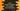# Java program to check if all digits of a number are in increasing order### Java program to check if all the digits of a number are in increasing order :

In this tutorial, we will learn how to check if all the digits of a number are in increasing order or not using Java. For example, for the number 12345 , all the digits are in increasing order. But for the number 54321, they are not in increasing order.

The user will first enter one number and our program will start scanning its digits from right to left. It will compare the rightmost element to the element left to it. For example, 1234, we will first compare 4 with 3. Then we will change the number to 123. Again compare the digit 3 to 2. If we found any rightmost number less than the left one, it will exit from the loop and print one failure message.

Let’s take a look at the Java program to check how to solve this problem :

### Java Program :

``````import java.util.Scanner;

class Main {
public static void main(String args[]) {

//1
int num;
boolean flag = false;

//2
Scanner scanner = new Scanner(System.in);

//3
System.out.println("Enter a number : ");
num = scanner.nextInt();

//4
int currentDigit = num % 10;
num = num/10;

//5
while(num>0){
//6
if(currentDigit <= num % 10){
flag = true;
break;
}

currentDigit = num % 10;
num = num/10;
}

//7
if(flag){
System.out.println("Digits are not in increasing order.");
}else{
System.out.println("Digits are in increasing order.");
}
}
}``````

### Explanation :

1. Create one integer variable num to store the user input number and one boolean flag to indicate if the numbers are in increasing or decreasing order.
2. Create one Scanner object to read the user input values.
3. Ask the user to enter a number. Read it and store it in num variable.
4. Create one integer currentDigit. It will hold the rightmost digit of the number. Convert the num to num/10.
5. Run one while loop to check for all digits of num.
6. If any right digit is less than the left digit, mark flag as true and break from the loop. Else, change the rightmost digit to the next left digit and change the number to number / 10.
7. Check the value of flag and print one message to the user. If flag is true, digits are not in increasing order, else they are in increasing order.

### Sample Output :

``````Enter a number :
123456
Digits are in increasing order.

Enter a number :
1234586
Digits are not in increasing order.

Enter a number :
1368
Digits are in increasing order.``````